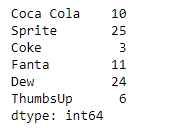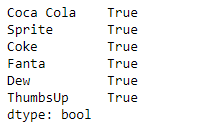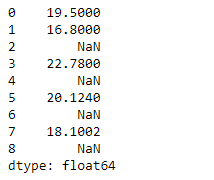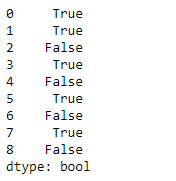# Python | Pandas Series.notna()

• Last Updated : 11 Feb, 2019

Pandas series is a One-dimensional ndarray with axis labels. The labels need not be unique but must be a hashable type. The object supports both integer- and label-based indexing and provides a host of methods for performing operations involving the index.

Pandas` Series.notna()` function Detect existing (non-missing) values. This function return a boolean object having the size same as the object, indicating if the values are missing values or not. Non-missing values get mapped to True. Characters such as empty strings ” or `numpy.inf` are not considered NA values (unless pandas.options.mode.use_inf_as_na = True is set). NA values, such as None or numpy.NaN, get mapped to False values.

Syntax: Series.notna()

Parameter : None

Returns : Series

Example #1: Use `Series.notna()` function to detect all the non-missing values in the given series object.

 `# importing pandas as pd``import` `pandas as pd`` ` `# Creating the Series``sr ``=` `pd.Series([``10``, ``25``, ``3``, ``11``, ``24``, ``6``])`` ` `# Create the Index``index_ ``=` `[``'Coca Cola'``, ``'Sprite'``, ``'Coke'``, ``'Fanta'``, ``'Dew'``, ``'ThumbsUp'``]`` ` `# set the index``sr.index ``=` `index_`` ` `# Print the series``print``(sr)`

Output :Now we will use `Series.notna()` function to detect the non-missing values in the series object.

 `# detect non-missing value``result ``=` `sr.notna()`` ` `# Print the result``print``(result)`

Output :As we can see in the output, the `Series.notna()` function has returned a boolean object. `True` indicates that the corresponding value is not missing. `False` value indicates that the value is missing. All the values are True in this series as there is no missing values.

Example #2: Use `Series.notna()` function to detect all the non-missing values in the given series object.

 `# importing pandas as pd``import` `pandas as pd`` ` `# Creating the Series``sr ``=` `pd.Series([``19.5``, ``16.8``, ``None``, ``22.78``, ``None``, ``20.124``, ``None``, ``18.1002``, ``None``])`` ` `# Print the series``print``(sr)`

Output :Now we will use `Series.notna()` function to detect the non-missing values in the series object.

 `# detect non-missing value``result ``=` `sr.notna()`` ` `# Print the result``print``(result)`

Output :As we can see in the output, the `Series.notna()` function has returned a boolean object. `True` indicates that the corresponding value is not missing. `False` value indicates that the value is missing.

My Personal Notes arrow_drop_up